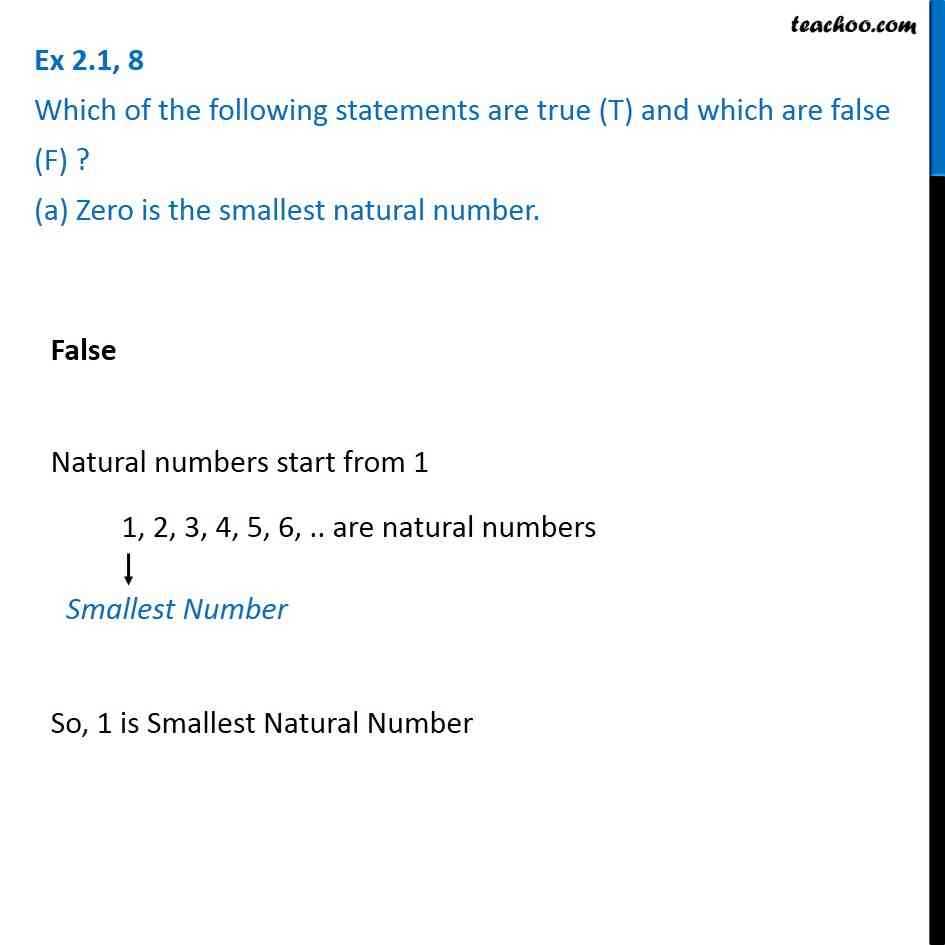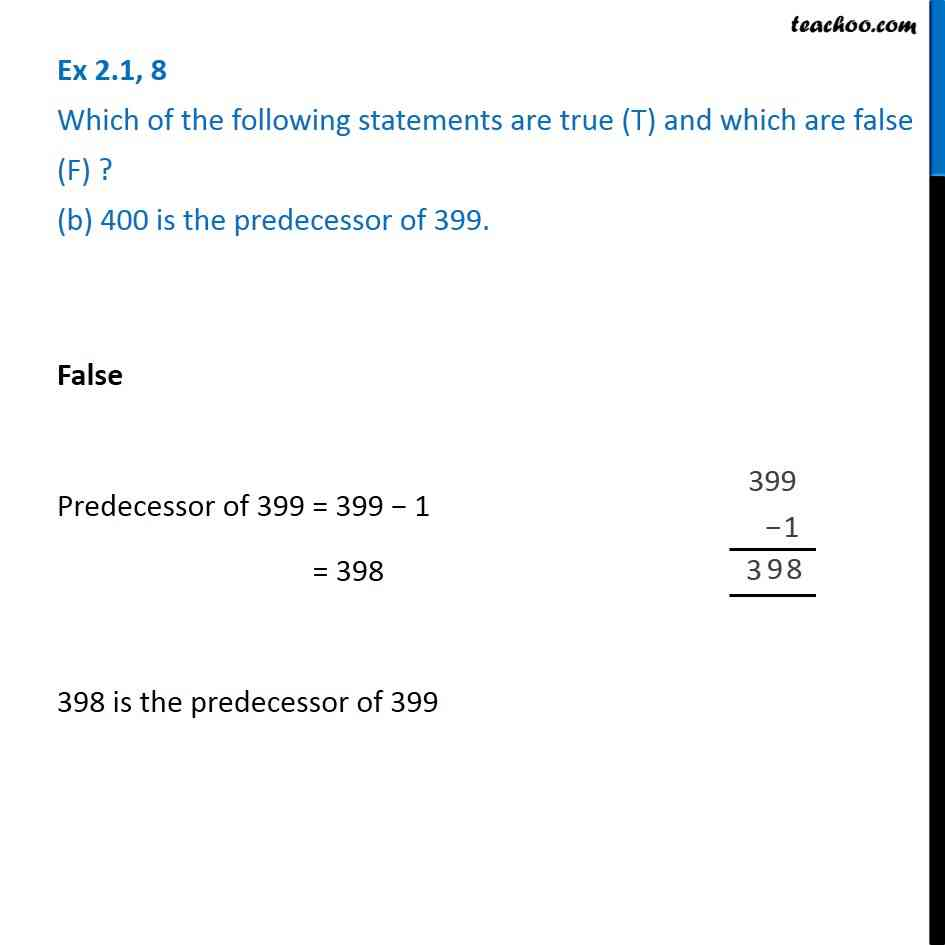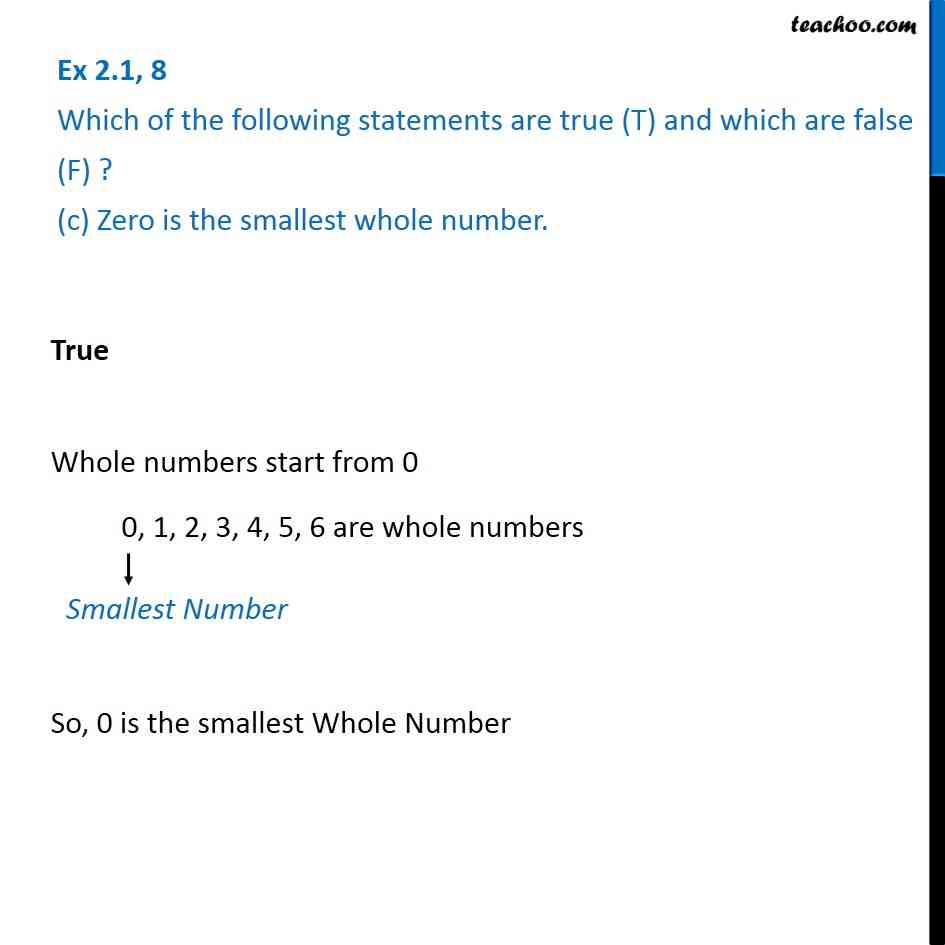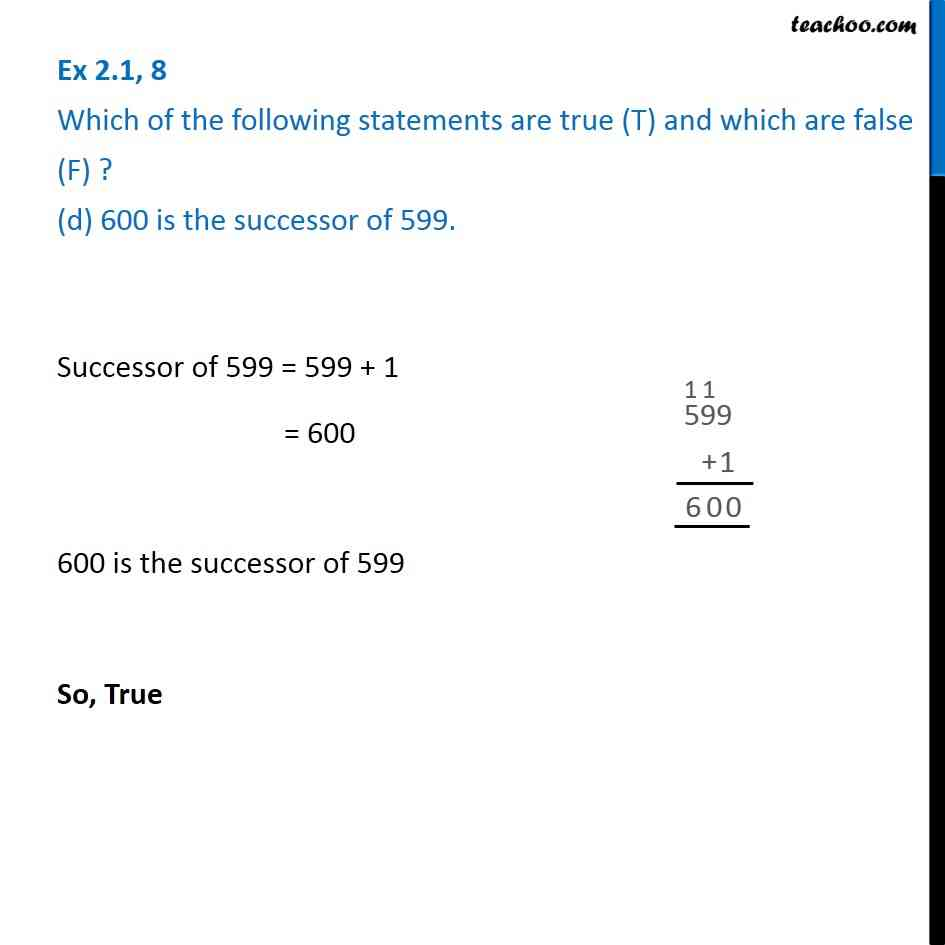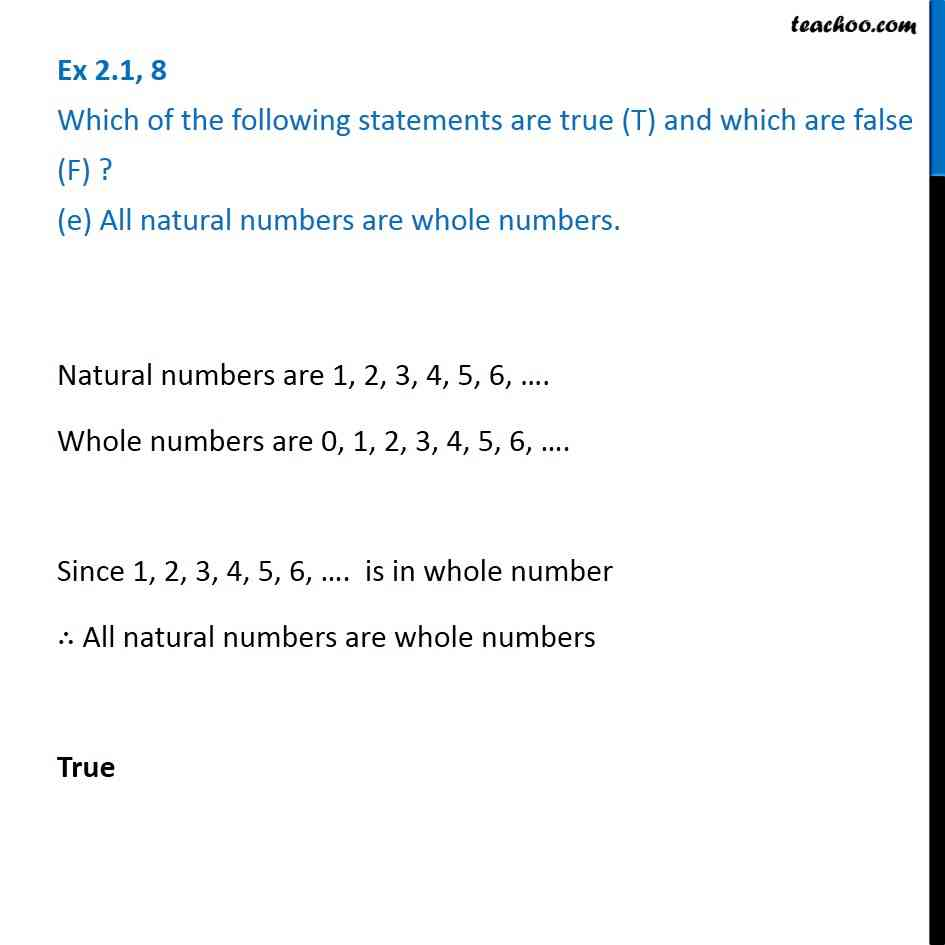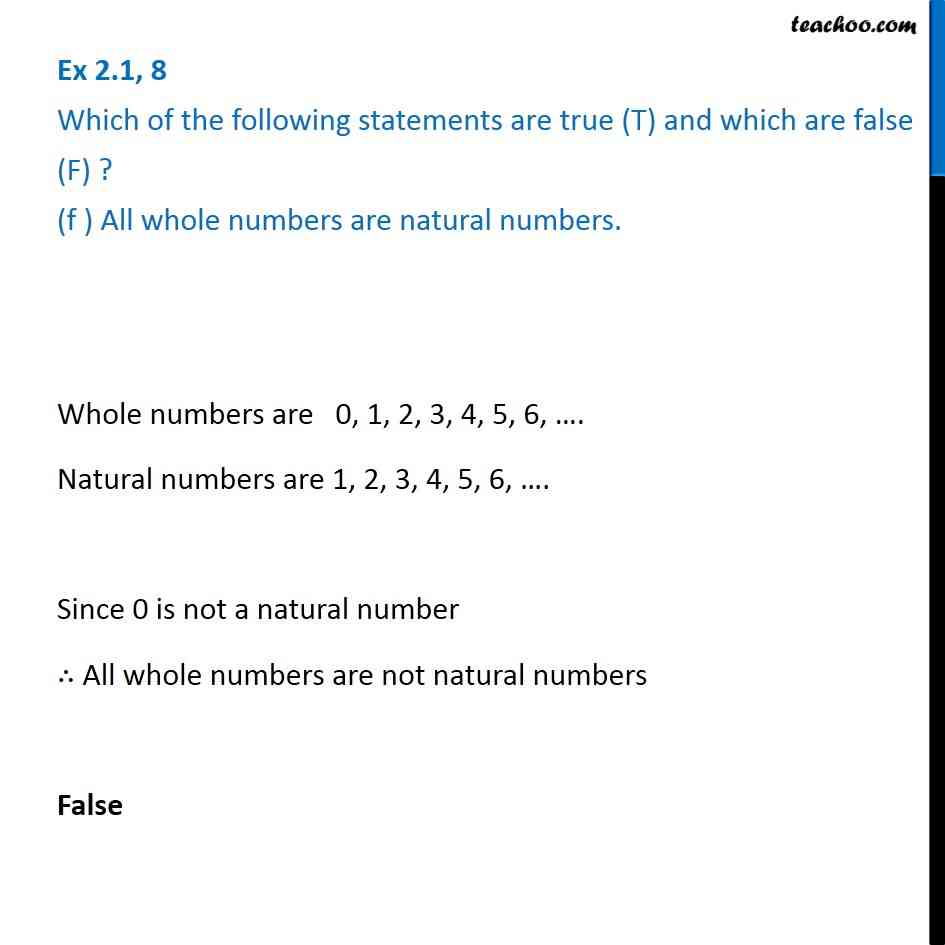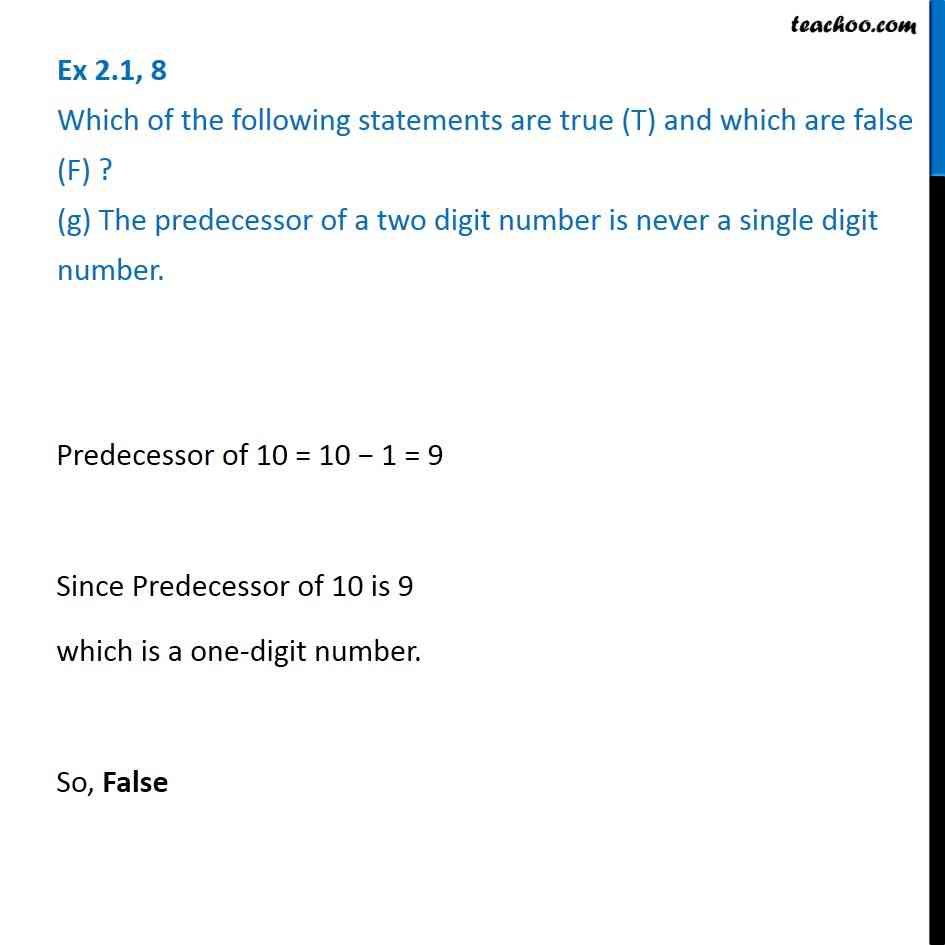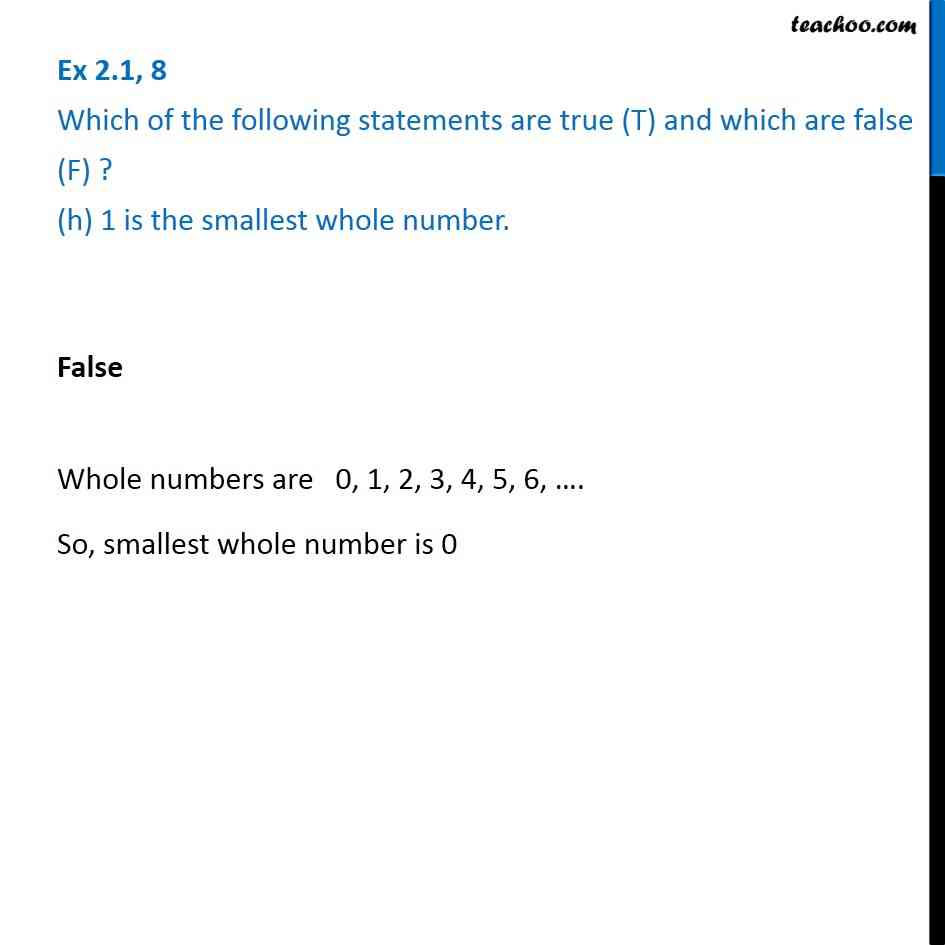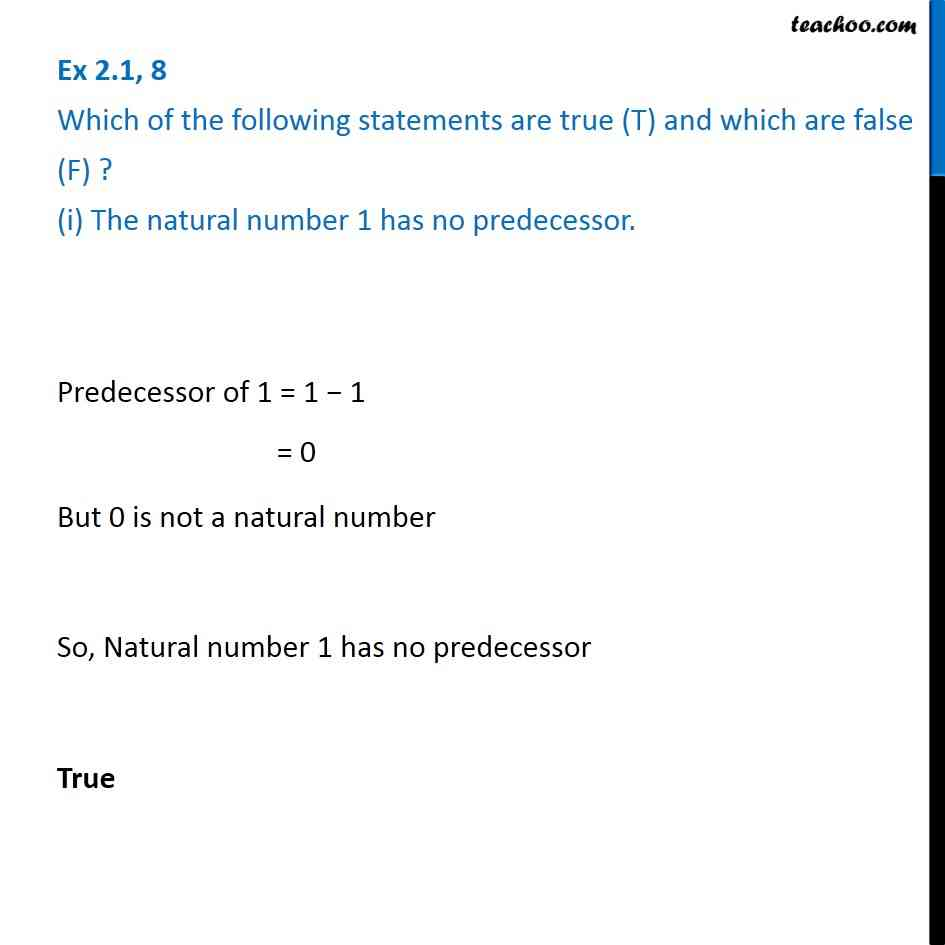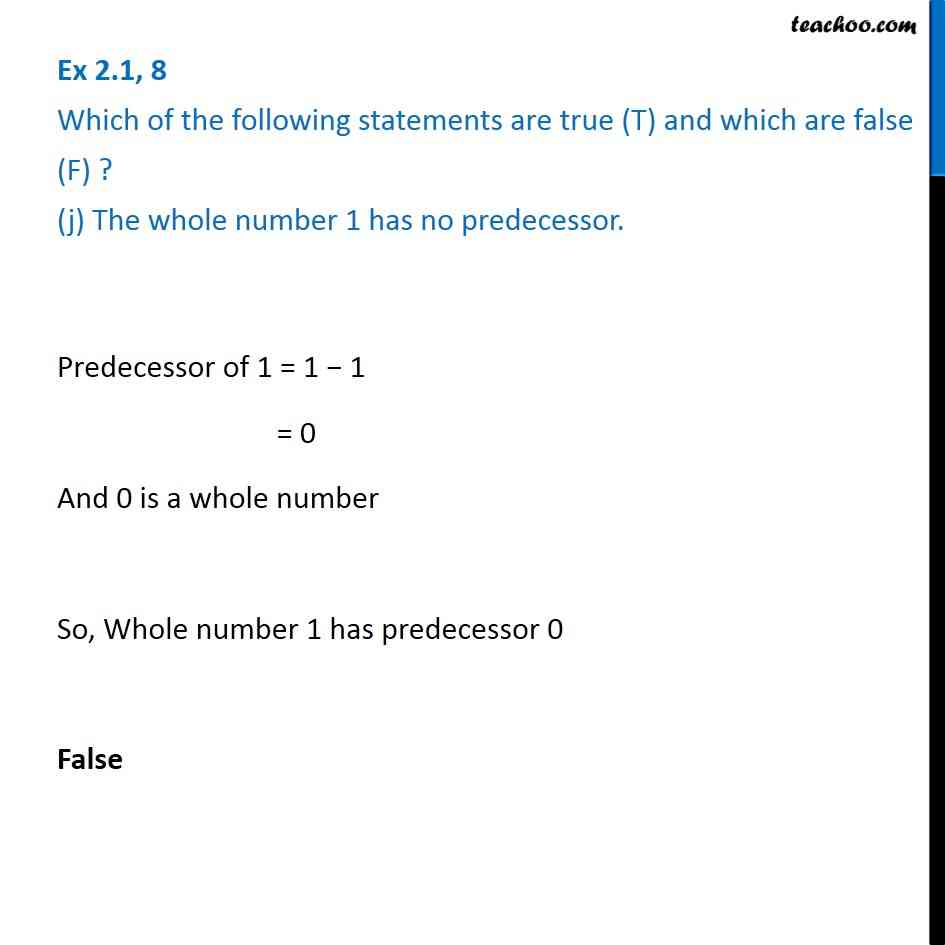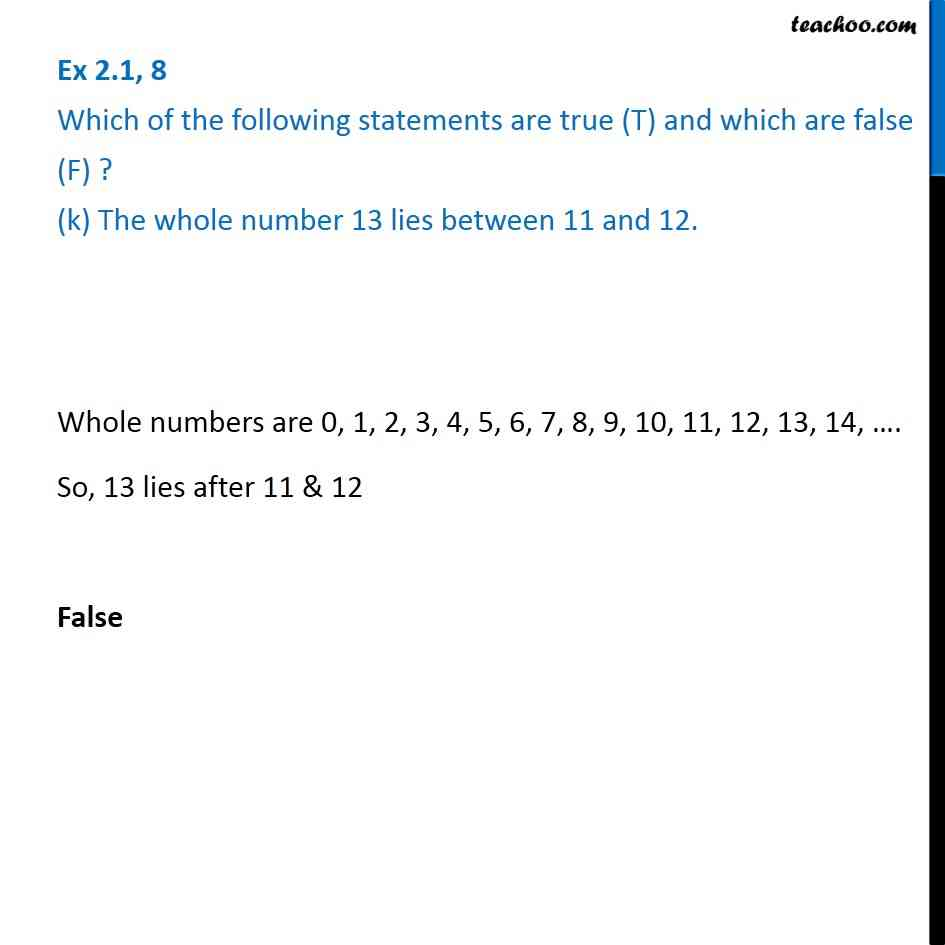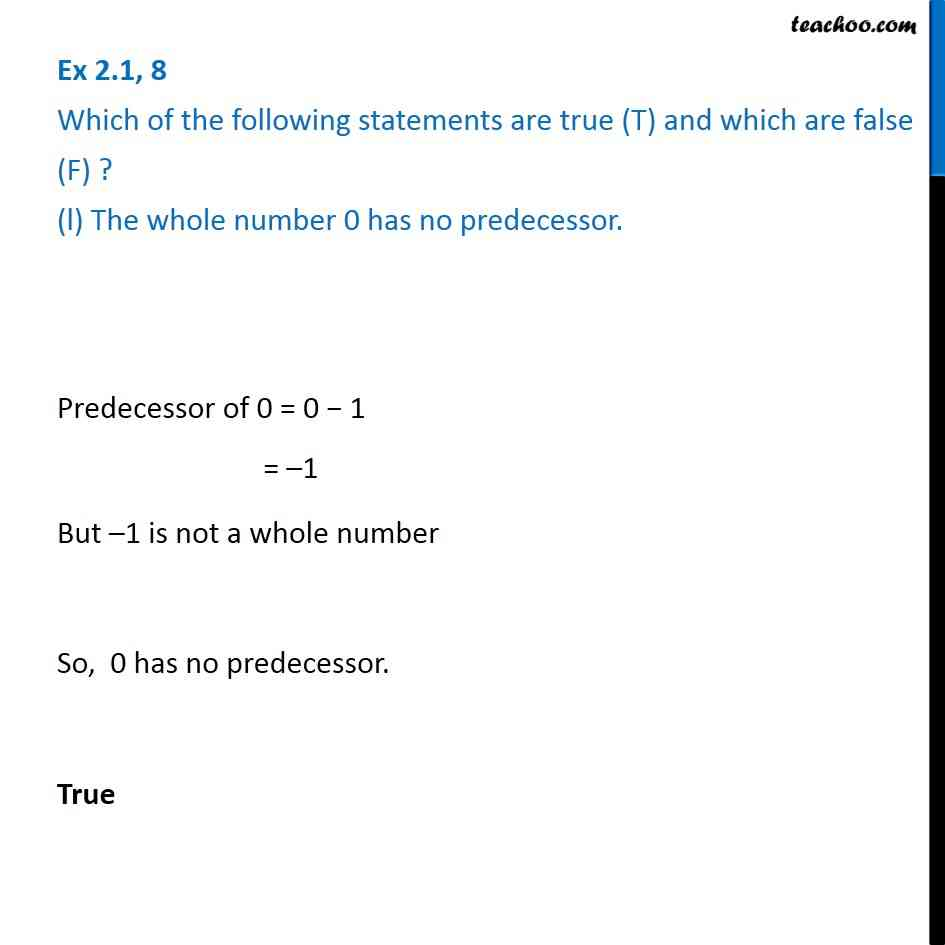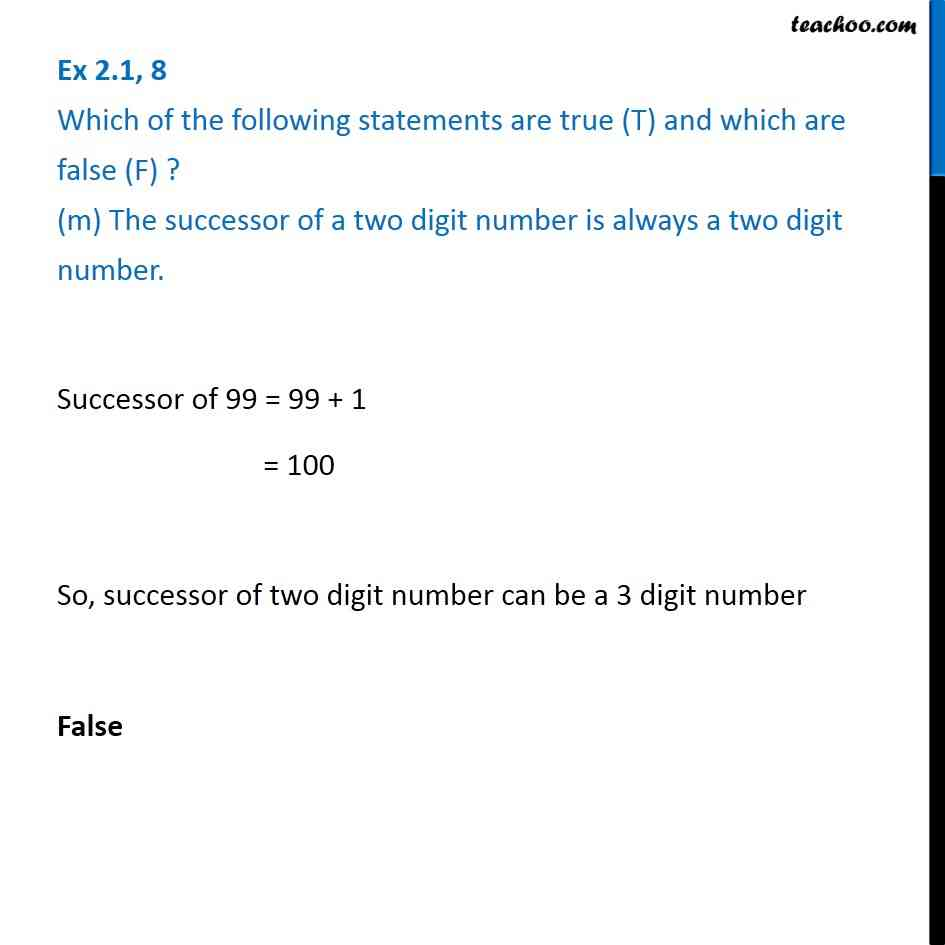Subscribe to our Youtube Channel - https://you.tube/teachoo

1. Chapter 2 Class 6 Whole Numbers
2. Serial order wise
3. Ex 2.1

Transcript

Ex 2.1, 8 Which of the following statements are true (T) and which are false (F) ? (a) Zero is the smallest natural number. False Natural numbers start from 1 1, 2, 3, 4, 5, 6, .. are natural numbers So, 1 is Smallest Natural Number Ex 2.1, 8 Which of the following statements are true (T) and which are false (F) ? (b) 400 is the predecessor of 399. False Predecessor of 399 = 399 - 1 = 398 398 is the predecessor of 399 Ex 2.1, 8 Which of the following statements are true (T) and which are false (F) ? (c) Zero is the smallest whole number. True Whole numbers start from 0 0, 1, 2, 3, 4, 5, 6 are whole numbers So, 0 is the smallest Whole Number Ex 2.1, 8 Which of the following statements are true (T) and which are false (F) ? (d) 600 is the successor of 599. Successor of 599 = 599 + 1 = 600 600 is the successor of 599 So, True Ex 2.1, 8 Which of the following statements are true (T) and which are false (F) ? (e) All natural numbers are whole numbers. Natural numbers are 1, 2, 3, 4, 5, 6, . Whole numbers are 0, 1, 2, 3, 4, 5, 6, . Since 1, 2, 3, 4, 5, 6, . is in whole number All natural numbers are whole numbers True Ex 2.1, 8 Which of the following statements are true (T) and which are false (F) ? (f) All whole numbers are natural numbers. Whole numbers are 0, 1, 2, 3, 4, 5, 6, . Natural numbers are 1, 2, 3, 4, 5, 6, . Since 0 is not a natural number All whole numbers are not natural numbers False Ex 2.1, 8 Which of the following statements are true (T) and which are false (F) ? (g) The predecessor of a two digit number is never a single digit number. Predecessor of 10 = 10 - 1 = 9 Since Predecessor of 10 is 9 which is a one-digit number. So, False Ex 2.1, 8 Which of the following statements are true (T) and which are false (F) ? (h) 1 is the smallest whole number. False Whole numbers are 0, 1, 2, 3, 4, 5, 6, . So, smallest whole number is 0 Ex 2.1, 8 Which of the following statements are true (T) and which are false (F) ? (i) The natural number 1 has no predecessor. Predecessor of 1 = 1 - 1 = 0 But 0 is not a natural number So, Natural number 1 has no predecessor True Ex 2.1, 8 Which of the following statements are true (T) and which are false (F) ? (j) The whole number 1 has no predecessor. Predecessor of 1 = 1 - 1 = 0 And 0 is a whole number So, Whole number 1 has predecessor 0 False Ex 2.1, 8 Which of the following statements are true (T) and which are false (F) ? (k) The whole number 13 lies between 11 and 12. Whole numbers are 0, 1, 2, 3, 4, 5, 6, 7, 8, 9, 10, 11, 12, 13, 14, . So, 13 lies after 11 & 12 False Ex 2.1, 8 Which of the following statements are true (T) and which are false (F) ? (l) The whole number 0 has no predecessor. Predecessor of 0 = 0 - 1 = -1 But -1 is not a whole number So, 0 has no predecessor. True Ex 2.1, 8 Which of the following statements are true (T) and which are false (F) ? (m) The successor of a two digit number is always a two digit number. Successor of 99 = 99 + 1 = 100 So, successor of two digit number can be a 3 digit number False

Ex 2.1